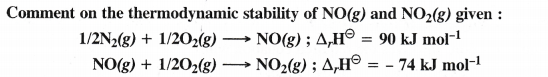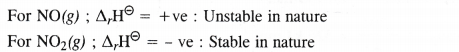## NCERT Solutions for Class 11 Chemistry Chapter 6 Thermodynamics

These Solutions are part of NCERT Solutions for Class 11 Chemistry. Here we have given NCERT Solutions for Class 11 Chemistry Chapter 6 Thermodynamics.

Question 1.
Choose the correct answer.
A thermodynamic state function is a quantity
(i) used to determine heat changes
(ii) whose value is independent of path
(iii) used to determine pressure volume work
(iv) whose value depends on temperature only.
(ii) whose value is independent of path

Question 2.
For the process to occur under adiabatic conditions, the correct condition is :
(i) ∆T = 0
(ii) ∆p = 0
(iii) q = 0
(iv) w = 0
(iii) q = 0

Question 3.
The enthalpies of all elements in their standard states are :
(i) unity
(ii) zero
(iii) < 0
(iv) different for each element
(ii) zero

Question 4.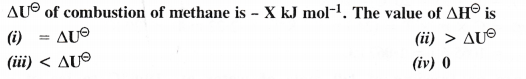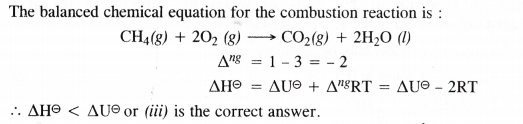Question 5.
The enthalpy of combustion of methane, graphite and dihydrogen at 298 K are – 890.3 kJ mol-1, -393.5 kJ mol-1 and – 285.8 kJ mol-1 respectively. Enthalpy of formation of CH4 (g) will be
(i) -74.8 kJ mol-1 (ii) -52.27 kJ mol-1
(iii) + 74.8 kJ mol-1 (iv) + 52.26 kJ mol-1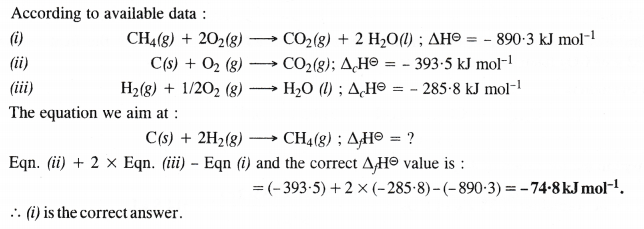Question 6.
A reaction, A + B → C + D + q is found to have a positive entropy change. The reaction will be
(i) possible at high temperature
(ii) possible only at low temperature
(iii) not possible at any temperature
(iv) possible at any temperature
(iv) possible at any temperature

Question 7.
In a process, 701 J of heat is absorbed by a system and 394 J of work is done by the system. What is the change in internal energy for the process ?
Heat absorbed by the system, q = 701 J
Work done by the system = – 304 J
Change in internal energy (∆U) = q + w = 701 – 394 = 307 J.

Question 8.
The reaction of cyanamide, NH2CN(s) with oxygen was affected in a bomb calorimeter and ∆U was found to be – 742.7 kJ mol-1 of cyanamide at 298 K. Calculate the enthalpy change for the reaction at 298 K.
NH2CN(y) + 3/2O2(g) → N2fe) + CO2(g) + H2O(l)
∆U = – 742.7 kJ mol-1 ; ∆ng = 2 – $$\frac { 3 }{ 2 }$$ = + $$\frac { 1 }{ 2 }$$ mol.
R = 8.314 × 10-3 kJ K-1 mol-1 ; T = 298 K
According to the relation, ∆H = ∆U + ∆ng RT
AH = (- 742.7 kJ) + ($$\frac { 1 }{ 2 }$$ mol) × (8.314 × 10-3 kJ K-1 mol-1) × (298 K)
= -742.7 kJ + 1.239 kJ = -741.5 kJ.

Question 9.
Calculate the number of kJ necessary to raise the temperature of 60 g of aluminium from 35 to 55°C. Molar heat capacity of Al is 24 J mol-1 K-1.
No. of moles of Al (m) = $$\frac { 60g }{ 27gmo{ l }^{ -1 } }$$ = 2.22 mol
Molar heat capacity (C) = 24 J mol-1 K-1
Rise in temperature (∆T) = 55 – 35 = 20°C = 20 K
Heat evolved (q) = C × m × T = (24 J mol-1 K-1) × (2.22 mol) × (20 K)
= 1065.6 J = 1.067 kJ.

Question 10.
Calculate the enthalpy change on freezing of 1.0 mole of water at – 10.0°C to ice at – 10.0°C. ∆fusH = 6.03 kJ mol-1 at 0°C; Cp[H2O(l)] = 75.3 J mol-1 K-1 ; Cp[H2O(s)l = 36.8 J mol-1 K-1.
Total change in enthalpy (AH) for the freezing process may be calculated as :
∆H = (1 male of water at 10°C → 1 mole of water at 0°C) + (1 mole of water at °C → 1 mole of ice at 0°C)
+ (1 mole of ice at °C → 1 mole of ice at – 10°C)
= Cp[H2O(l)] × ∆T + ∆Hfreezmg + Cp [H2O(s)] × ∆T.
= (75.3 Jk-1 mol-1) (0 – 10 K) + (-6.03 kJ mol-1) + (36.8Jk-1 mol-1) × (-10 K)
= (-753 J mol-1) – (6.03 kJ mol-1) – (368 J mol-1)
= (-0.753 kJ mol-1) – (6.03 kJ mol-1) – (0.368 kJ mol-1)
= – 7.151 kJ mol-1

Question 11.
Enthalpy of combustion of carbon to carbon dioxide is – 393.5 kJ mol-1. Calculate the heat released upon formation of 35.2 g of CO2 from carbon and oxygen gas.
The combustion equation is :
C(s) + O2(g) → CO2(g) ; ∆cH = – 393.5 kJ mol-1 (44 g)
(44g)
Heat released in the formation of 44g of CO2 = 393.5 kJ
Heat released in the formation of 35.2 g of CO2 = $$\frac { (393.5kJ)\times (35.2g) }{ (44g) }$$ = 314.8 kJ.

Question 12.
Calculate the enthalpy of the reaction :
N2O4(g) + 3CO(g) → N2O(g) + 3CO2(g)
Given that;
fH CO(g) = – 110 kJ mol-1 ;
fH CO2(g) = – 393 kJ mol-1
fH. N2O(g) = 81 kJ mol-1 ;
fH N2O4(g) = – 9.7 kJ mol-1.
Enthalpy of reaction (∆rH) = [81 + 3(- 393)] – [9.7 + 3(- 110)]
= [81 – 1179] – [9.7 – 330] = – 778 kJ mol-1.

Question 13.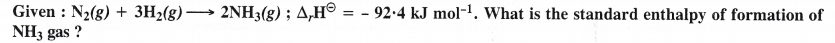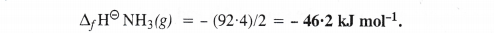Question 14.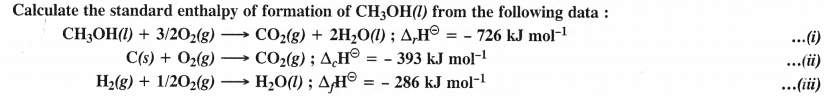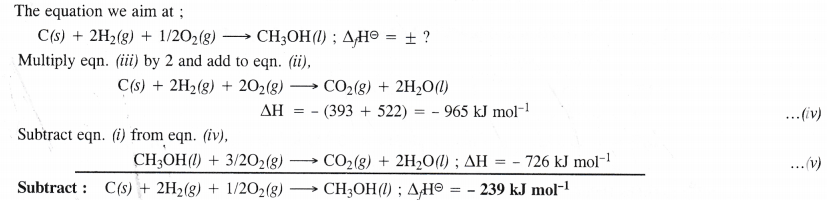Question 15.
Calculate the enthalpy change for the process
CCl4 (g) → C (g) + 4 Cl (g) and calculate bond enthalpy of C—Cl in CC14 (g)
Given : ∆vap H° (CCl4) = 30.5 kJ mol-1 ; ∆fH°(CCl4) = – 135.5 kJ mol-1
aH° (C) = 715.0 kJ mol-1 where ∆a H° is enthalpy of atomisation
aH° (Cl2) = 242 kJ mol-1.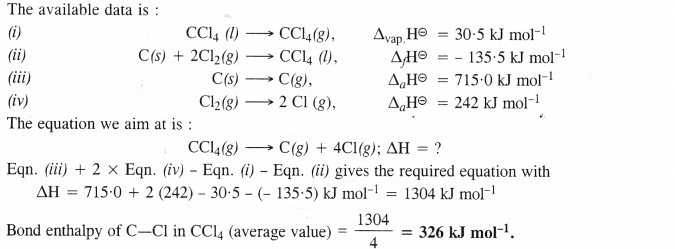Question 16.
For an isolated system ∆U = 0 ; what will be ∆S ?
Change in internal energy (∆U) for an isolated system is zero because it does not exchange any energy with the surroundings. But entropy tends to increase in case of spontaneous reaction. Therefore, ∆S > 0 or positive.

Question 17.
For a reaction at 298 K
2 A + B → C
∆H = 400 kJ mol-1 and ∆S = 0.2 kJ K’1 mol-1.
At what temperature will the reaction become spontaneous considering ∆H and ∆S to be constant over the temperature range ?
According to Gibbs-Helmholtz equation :
∆G = ∆H – T∆S
For ∆G = 0; ∆H = T∆S or T : = $$\frac { \triangle H }{ \triangle s }$$

T = $$\frac { (400kJmo{ l }^{ -1 }) }{ (0.2kJ{ K }^{ -1 }mo{ l }^{ -1 }) }$$
Thus, reaction will be in a state of equilibrium at 2000 K and will be spontaneous above this temperature.

Question 18.
For the reaction ; 2Cl (g) → Cl2(g) ; what will be the signs of ∆H and ∆S ?
∆H : negative (-ve) because energy is released in bond formation
∆S : negative (-ve) because entropy decreases when atoms combine to form molecules.

Question 19.
For a reaction ; 2A (g) + B (g) → 2D(g)Calculate ∆U298 for the reaction and predict whether the reaction is spontaneous or not.
Let the mass of H2 in the mixture = 20 g
The mass of O2 in the mixture will be = 80 g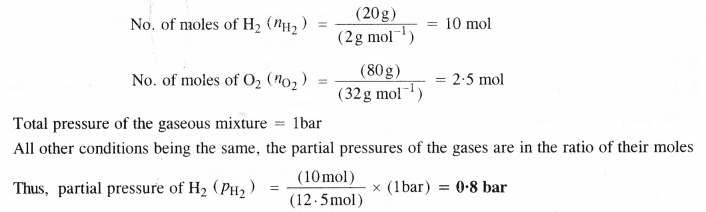Question 20.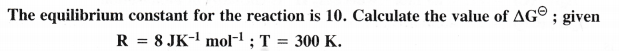Question 21.